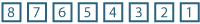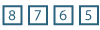## Content

### Expansions and the notation $$\binom{n}{r}$$

#### Expansions

We start by looking at the results of multiplying several binomials. With two binomials, we have

$(a+b)(c+d) = a(c+d) + b(c+d) = ac+ad+bc+bd.$

The expansion is obtained by multiplying each letter in the first bracket by each letter in the second and adding them. There are $$2\times 2= 2^2 =4$$ terms. Similarly, with three binomials, we have

$(a+b)(c+d)(e+f) = ace+acf+ade+adf+bce+bcf+bde+bdf.$

There are $$2\times2\times 2 = 2^3 =8$$ terms.

##### Exercise 1
1. How many terms are there in the expansion of $$(a+b+c)(d+e)$$?
2. How many terms are there in the expansion of $$(a+b)(c+d)(e+f)(g+h)(i+j)$$?

In general, the product of any number of polynomials is equal to the sum of all the products which can be formed by choosing one term from each polynomial and multiplying these terms together.

#### Example

Find the coefficient of $$x^2$$ in the expansion of $$(2x-1)(3x+4)(5x-6)$$.

#### Solution

If we take the terms containing $$x$$ from any two of the factors and the constant from the remaining factor and multiply these terms together, we will obtain a term containing $$x^2$$ in the expansion. If we do this in all possible ways and add, we will find the required coefficient.

The required coefficient is

\begin{align*} 2 \times 3\times(-6)+3\times 5\times(-1)+2\times 5\times 4 &=-36-15+40\\ &=-11. \end{align*}

#### Permutations and factorial notation

In how many ways can eight people line up to get into a theme-park ride?

We can draw a box diagram for this situation, with each box indicating the number of choices for each position.So the answer is $$8\times 7\times 6\times 5\times 4\times 3\times 2\times 1 = 40\,320$$ ways. The notation for this is $$8!$$. This is read as eight factorial.

Most calculators have a factorial key.

The number of ways to arrange $$n$$ different objects in a row is $$n!$$. It is important to note that $$n!=n(n-1)!$$. For this statement to be true when $$n=1$$, we define $$0! = 1$$.

If there are eight competitors in a race, in how many ways can the first four places be filled? We can draw a box diagram for this situation, with each box indicating the number of choices for each position.So the answer is $$8\times 7\times 6\times 5 = 1680$$ ways.

A permutation is an arrangement of elements chosen from a certain set. For example, consider the set $$\{a,b,c,d,e\}$$. Some of the permutations of three letters taken from this set include

$abc, \qquad bac, \qquad cab, \qquad eda.$

Altogether, there are $$5\times 4\times 3 = 60$$ such permutations.

The symbol $${^n}P_r$$ is used to denote the number of permutations of $$r$$ distinct objects chosen from $$n$$ objects. We see that

${^n}P_r =n(n-1)\dotsm(n-r+1)=\dfrac{n!}{(n-r)!}.$

#### Example

List all the permutations of the letters in the word CAT.

#### Solution

There are $$3 \times 2 \times 1 = 6$$ such permutations: CAT, CTA, TAC, TCA, ACT, ATC.

#### Example

Seven runners are competing in a race. In how many ways can the gold, silver and bronze medals be awarded?

#### Solution

The gold medal can be awarded in 7 ways. The silver medal can then be awarded in 6 ways. The bronze medal can then be awarded in 5 ways. The total number of ways of awarding the medals is $$7\times 6\times 5 = 210$$ ways.

#### The notation $$\binom{n}{r}$$

Let us return to the set of five letters $$\{a,b,c,d,e\}$$, but this time we are only interested in choosing three of the letters with no concern for order. The three letters $$a,b,c$$ can be arranged in six ways:

$abc, \qquad acb, \qquad bac, \qquad bca, \qquad cab, \qquad cba.$

Similarly, any group of three letters can be arranged in six ways. The number of permutations of three letters chosen from five letters is $${^5}P_3 = 5 \times 4 \times 3 = 60$$. Therefore the number of ways of choosing three letters from five letters is $$\dfrac{60}{6} = 10$$. We list them here:

Remember that each set of three elements can be arranged in six ways.

We denote the number of ways of choosing $$r$$ objects from $$n$$ objects by $$\dbinom{n}{r}$$, which is read as '$$n$$ choose $$r$$'.

In the example above, we found that

$\dbinom{5}{3} = \dfrac{{^5}P_3}{3!} = \dfrac{60}{6} = 10.$

In general, we have

$\dbinom{n}{r} = \dfrac{^nP_r}{r!} = \dfrac{n!}{(n-r)!r!}.$

Consider the five-element set $$\{a,b,c,d,e\}$$ again. There are

\begin{alignat*}{2} \dbinom{5}{1}&=5 \text{ ways of choosing 1 letter},&\qquad \dbinom{5}{2}&=10 \text{ ways of choosing 2 letters},\\ \dbinom{5}{3}&=15 \text{ ways of choosing 3 letters},&\qquad \dbinom{5}{4}&=10 \text{ ways of choosing 4 letters},\\ \dbinom{5}{5}&=1 \text{ way of choosing 5 letters}. \end{alignat*}

Listing these as a row, including choosing no letters as the first entry:

$\dbinom{5}{0}=1, \qquad \dbinom{5}{1}=5, \qquad \dbinom{5}{2}=10, \qquad \dbinom{5}{3}=10, \qquad \dbinom{5}{4}=5, \qquad \dbinom{5}{5}=1.$

This is the fifth row of Pascal's triangle.

#### Example

Evaluate

1. $$\dbinom{100}{2}$$
2. $$\dbinom{1000}{998}$$.

#### Solution

1. $$\dbinom{100}{2} = \dfrac{100\times99}{2} = 4950$$
2. $$\dbinom{1000}{998} = \dfrac{1000\times999}{2} = 499\,500$$.

#### Example

In how many ways can you choose two people from a group of seven people?

#### Solution

There are $$\dbinom{7}{2} = \dfrac{7\times6}{2} = 21$$ ways of choosing two people from seven.

#### Example

There are ten people in a basketball squad. Find how many ways:

1. the starting five can be chosen from the squad
2. the squad can be split into two teams of five.

#### Solution

1. There are $$\dbinom{10}{5} = \dfrac{10\times 9\times 8\times7\times6}{5\times4\times3\times2\times1} = 252$$ ways of choosing the starting five.
2. The number of ways of dividing the squad into two teams of five is $$\dfrac{252}{2} = 126$$.

If we expand $$(a+b)^6$$, we know the terms will be of the form

$c_0 a^6, \qquad c_1a^5b, \qquad c_2a^4b^2, \qquad c_3a^3b^3, \qquad c_4a^2b^4, \qquad c_5ab^5, \qquad c_6 b^6,$

where $$c_i$$ are the coefficients. With

$(a+b)^6 = (a+b)(a+b)(a+b)(a+b)(a+b)(a+b),$

we can use combinations to find the coefficients.

For $$c_5$$, the relevant terms when multiplying out are

$abbbbb, \qquad babbbb, \qquad bbabbb, \qquad bbbabb, \qquad bbbbab, \qquad bbbbba.$

There are $$\dbinom{6}{1}=6$$ ways of choosing one $$a$$ from the six brackets. Equivalently, there are $$\dbinom{6}{5}=6$$ ways of choosing five $$b$$'s from the six brackets. Therefore $$c_5 = 6$$.

We can find the values of the other coefficients in the same way. This is done in the following example.

#### Example

Write out the expansion of $$(a+b)^{10}$$.

#### Solution

The terms are

\begin{alignat*}{6} & a^{10}, \qquad && c_1a^9b, \qquad && c_2a^8b^2, \qquad && c_3a^7b^3, \qquad && c_4a^6b^4, \qquad && c_5a^5b^5,\\ & c_6a^4b^6 , \qquad && c_7a^3b^7 , \qquad && c_8a^2b^8, \qquad && c_9ab^9, \qquad && b^{10}, \end{alignat*}

where $$c_i$$ are the coefficients.

Using a similar argument to that given above, we have

$c_1=\dbinom{10}{1}, \qquad c_2=\dbinom{10}{2}, \qquad c_3=\dbinom{10}{3}, \qquad \dots.$

Therefore

\begin{align*} (a+b)^{10} = a^{10}& \ +10a^9b+45a^8b^2+120a^7b^3+210a^6b^4+252a^5b^5\\ & \ +210a^4b^6+120a^3b^7+45a^2b^8+10ab^9+b^{10}. \end{align*}
##### Exercise 2

Let $$c_i$$ denote the coefficient of the term $$a^{12-i}b^i$$ in the expansion of $$(a+b)^{12}$$.

Write down the values of the coefficients $$c_2$$, $$c_3$$, $$c_5$$ and $$c_9$$ using the notation $$\dbinom{12}{r}$$, and evaluate each of these coefficients.

Interactive 1Next page - Content - The binomial theorem